×#### Thank you for registering.

One of our academic counsellors will contact you within 1 working day.

Click to Chat

1800-1023-196

+91-120-4616500

CART 0

• 0

MY CART (5)

Use Coupon: CART20 and get 20% off on all online Study Material

ITEM
DETAILS
MRP
DISCOUNT
FINAL PRICE
Total Price: Rs.

There are no items in this cart.
Continue Shopping• Complete Physics Course - Class 11
• OFFERED PRICE: Rs. 2,968
• View Details

```Reflection at Spherical Surfaces

Table of Content

Reflection at Curved Surfaces

Images formed by a Spherical Mirror

Image formed by a Convex Mirror

Cartesian Sign Convention

Relation between u, v and f for Spherical Mirrors

Related Resources

Reflection at Curved Surfaces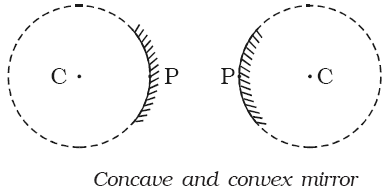In optics we are mainly concerned  with curved mirrors which are the part of a hollow sphere as shown in figure. One surface of the mirror is silvered. Reflection takes place at the other surface. If the reflection takes place at the concave surface, (which is towards the centre of the sphere) it is called concave mirror. If the reflection takes place at the convex surface, (which is away from the centre of the sphere) it is called convex mirror. The laws of reflection at a plane mirror are equally true for spherical mirrors also.

The centre of the sphere, of which the mirror is a part is called the centre of curvature (C). The geometrical centre of the mirror is called its pole (P). The line joining the pole of the mirror and its centre of curvature is called the principal axis. The distance between the pole and the centre of curvature of the spherical mirror is called the radius of curvature of the mirror and is also equal to the radius of the sphere of which the mirror forms a part.

When a parallel beam of light is incident on a spherical mirror, the point where the reflected rays converge (concave mirror) or appear to diverge from the point (convex mirror) on the principal axis is called the principal focus (F) of the mirror. The distance between the pole and the principal focus is called the focal length (f) of the mirror as shown in figure.Images formed by a Spherical Mirror

The images produced by spherical mirrors may be either real or virtual and may be either larger or smaller than the object. The image can be located by graphical construction as shown in figure by adopting any two of the following rules.

(a) A ray parallel to the principal axis after reflection by a concave mirror passes through the principal focus of the concave mirror and appear to come from the principal focus in a convex mirror.

(b) A ray passing through the centre of curvature retraces its path after reflection.

(c) A ray passing through the principal focus, after reflection is rendered parallel to the principal axis.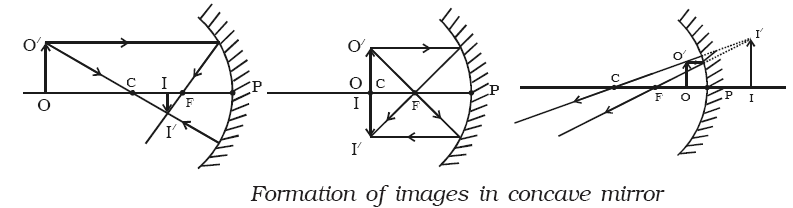(d) A ray striking the pole at an angle of incidence i is reflected at the same angle i to the axis.Image formed by a Convex Mirror

In a convex mirror irrespective of the position of the object, the image formed is always virtual, erect but diminished in size. The image lies between the pole and the focus (Fig. 9.10).

In general, real images are located in front of a mirror while virtual images behind the mirror.

Cartesian Sign Convention

The following sign conventions are used.

(a) All distances are measured from the pole of the mirror (in the case of lens from the optic centre).(b) The distances measured in the same direction as the incident light, are taken as positive.

(c) The distances measured in the direction opposite to the direction of incident light are taken as negative.

(d) Heights measured perpendicular to the principal axis, in the upward direction are taken as positive.

(e) Heights measured perpendicular to the principal axis, in the downward direction are taken as negative.

(f) The size of the object is always taken as positive, but image size is positive for erect image and negative for an inverted image.

(g) The magnification is positive for erect (and virtual) image, and negative for an inverted (and real) image.

Watch this Video for more reference

Relation between u, v and f for Spherical Mirrors

A mathematical relation between object distance u, the image distance v and the focal length f of a spherical mirror is known as mirror formula.

(a) Concave Mirror - Real Image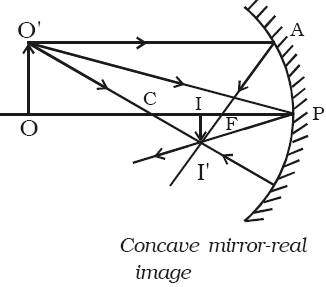Let us consider an object OO′ on the principal axis of a concave mirror beyond C. The incident and the reflected rays are shown in the figure. A ray O′A parallel to principal axis is incident on the concave mirror at A, close to P. After reflections the ray passes through the focus F. Another ray O′C passing through centre of curvature C, falls normally on the mirror and reflected back along the same path. A third ray O′P incident at the pole P is reflected along PI′. The three reflected rays intersect at the point I′. Draw perpendicular I′I to the principal axis. II′ is the real, inverted image of the object OO′.

Right angled triangles, II ′P and OO′P are similar

So, II′/OO′ = PI/PO

Right angled triangles II′ F and APF are also similar (A is close to P; hence AP is a vertical line)

Thus, II′/AP = IF/PF

AP = OO′ . Therefore the above equation becomes,

II′/ OO′ = IF/PF

Comparing the equations (1) and (2)

PI/PO = IF/PF

But, IF = PI – PF            …... (3)

Therefore equation (3) becomes,

PI/PO = PI – PF/PF          …... (4)

Using sign conventions, we have PO = -u,

PI = -v and PF = -f

Substituting the values in the above equation, we get

-v/-u = -v – (-f)/-f      (or)

v/u = v – f/f = v/f – 1

Dividing by v and rearranging,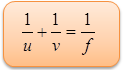This is called mirror equation. The same equation can be obtained for virtual image also.

(b) Convex Mirror - Virtual Image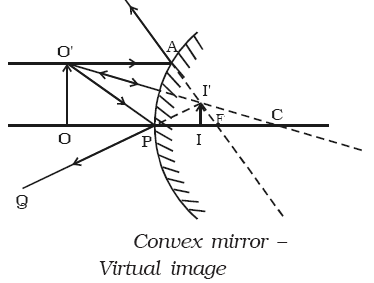Let us consider an object OO′ anywhere on the principal axis of a convex mirror. The incident and the reflected  rays  are  shown  in  the figure. A ray O′A parallel to the principal axis incident on the convex mirror at A close to P. After reflection the ray appears to diverge from the focus F. Another ray O′C passing through centre of curvature C, falls normally on the mirror and is reflected back along the same path. A third ray O ′P incident at the pole P is reflected along PQ. The three reflected rays when produced appear to meet at the point I ′. Draw perpendicular II′ to the principal axis. II′ is the virtual image of the object OO′.

Right angled triangles, II ′P and OO ′P are similar.

So,  II ′/OO ′ = PI/PO               …... (1)

Right angled triangles  II ′F and APF are also similar (A is close to P; hence AP is a vertical line)

II ′/AP = IF/PF

AP = OO ′. Therefore the above equation becomes,

II ′/OO ′ = IF/PF             …... (2)

Comparing the equations (1) and (2), we get,

PI / PO = IF/PF

But, IF = PF – PI. Therefore equation (3) becomes,

PI/PO = PF – PI/PF

Using sign conventions, we have PO = -u, PI = +v and PF = +f. Substituting the values in the above equation, we get

+v/-u = +f – (+v)/+f

or, – (v/u) = f – v/f = 1 – (v/f)

Dividing by v and rearranging we get,This is called mirror equation for convex mirror producing virtual image.A curved mirror formed by a part of a hollow glass sphere with a reflecting surface (created by depositing silver metal) is also referred to as a spherical mirror.

Curved mirrors have curved surfaces that reflect the light rays.

The curved surfaces are parts of spherical surfaces and hence they are also called as spherical mirrors.

The reflecting surface may be bulged towards the object or concave right to the object.

Curved mirrors have extensive uses in various applications.

Most curved mirrors have surfaces that are shaped like part of a sphere, but other shapes are sometimes used in optical devices.

A convex mirror or diverging mirror, is a curved mirror in which the reflective surface bulges toward the light source.

Convex mirrors reflect light outwards, therefore they are not used to focus light.

Convex mirrors always form a virtual, since the focus (F) and the centre of curvature (2F) are both imaginary points "inside" the mirror, which cannot be reached.

Images formed by these mirrors cannot be projected on a screen, since the image is inside the mirror.The image is smaller than the object, but gets larger as the object approaches the mirror.

Concave mirrors reflect light inward to one focal point.They are used to focus light.

Unlike convex mirrors, concave mirrors show different image types depending on the distance between the object and the mirror.

Concave mirrors are called "converging" because they tend to collect light that falls on them, refocusing parallel incoming rays toward a focus.

Problem (JEE Main):

If the distances of an object and its virtual image from the focus of a convex lens of focal length f are 1 cm each, then f is

(a) 4 cm      (b) (√2 + 1) cm

(c) 2√2 cm   (d) (2+√2) cm

Solution:

Here, u = – (f – 1)

v = – (f+1)

f = +f

Applying 1/v – 1/u = 1/f, we have,

[1/-(f+1)] + [1/(f-1)] = 1/f, we have,

[1/- (f+1)] + [1/(f –1)] = 1/f

Or, f2 – 2f – 1 = 0

This gives, f = (√2 + 1) cm

Thus from the above observation we conclude that, option (b) is correct.

Problem:

A ray of light on a plane mirror along a vector i+j+k. The normal on incident point is along i+j . Find a unit vector along the reflected ray?

Solution:

Reflection of a ray of light is just like an elastic collision of a ball with a horizontal ground. Component of incident ray along the inside normal gets reversed while the component perpendicular to it remains unchanged. Thus the

component of incident ray vector  A = i+j+k parallel to normal, i.e i+j,  gets reversed while perpendicular to it, i.e. -k  remains unchanged. Thus, the reflected ray can be written as

R = -i -j -k

A unit vector along the reflected ray will be

r = R/ |R| = (-i -j -k)/√3Question 1

Light passes through a closed tube which contains a gas. If the gas inside the tube is gradually pumped out, the speed of light inside the tube

(a) increases                         (b) decreases

(c) remains constant               (d) first increases and then decreases

Question 2

A converging lens is used to form an image on a screen. When the lower half of the lens is covered by an opaque screen then,

(a) half of the image will disappear

(b) complete image will be formed

(c) no image is formed

(d) intensity of the image is high

Question 3

An image formed by a convex mirror is always ________.

(a) virtual, erect and diminished

(b) virtual, real and magnified

(c) real, inverted and diminished

(d) real, erect and magnified

Question 4

Image formed by a concave mirror is erect and enlarged.What is the position of the object?

(a) Between focus F and the center of curvature

(b) At the center of curvature

(c) Beyond the center of curvature

(d) Between pole and the focus

Question 5

Center of curvature is not a part of spherical mirror rather it lies ----------- the mirror.

(a) boundary        (b) inside

(c) outside           (d) none of the aboveQ.1
Q.2
Q.3
Q.4
Q.5

a

b

a

d

c

Related Resources

You might like to magnification and thin lens.

For getting an idea of the type of questions asked, refer the  Previous Year Question Papers.

To read more, Buy study materials of Ray Optics and Optical Instruments comprising study notes, revision notes, video lectures, previous year solved questions etc. Also browse for more study materials on Physics here.
```### Course Features

• 101 Video Lectures
• Revision Notes
• Previous Year Papers
• Mind Map
• Study Planner
• NCERT Solutions
• Discussion Forum
• Test paper with Video Solution